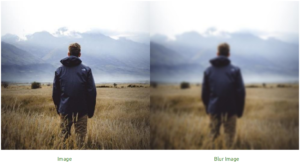Open In App

# Wand – blur() function in Python

Blurring Image means making an image fuzzy or hazy. A blur image is indefinite and it is unable to see an image clearly. Blur is of many types, like – Adaptive blur, Gaussian blur, Selective Blur etc. In order to blur an image we use `blur()` function. `blur()` function takes three arguments.

Example :Syntax :

 `wand.image.blur(radius``=``"radius_value"``, ``                  ``sigma``=``"sigma_value"``,  ``    ``channel ``=` `"optional_channel_value"``)`

Parameters :

ParameterInput TypeDescription
radiusnumbers.realthe radius of the, in pixels, not counting the center pixel. Default is 0.0.
sigmanumbers.realthe standard deviation of the, in pixels. Default value is 0.0.
channelbasestringOptional color channel to apply blur.

Example #1:

Input Image –`# import Image from wand.image module``from` `wand.image ``import` `Image`` ` `# read file using Image function``with Image(filename ``=``"koala.jpeg"``) as img:`` ` `    ``# perform blur effect using blur() function``    ``img.blur(radius ``=` `0``, sigma ``=` `3``)`` ` `    ``# save final image``    ``img.save(filename ``=``"blur_koala.jpeg"``)`

Output:Example #2:

Input Image –`# import Image from wand.image module``from` `wand.image ``import` `Image``from` `wand.display ``import` `display`` ` `# read file using Image function``with Image(filename ``=``"human1.jpeg"``) as img:`` ` `    ``# perform blur effect using blur() function``    ``img.blur(radius ``=` `3``, sigma ``=` `4``, )`` ` `    ``# save final image``    ``img.save(filename ``=``"blurhuman.jpeg"``)``    ``display(img)`

Output: